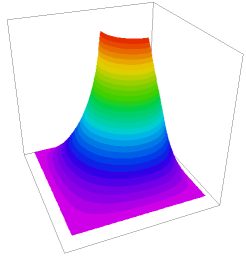﻿ Sample Problems > Applications > Misc > minimal_surface

# minimal_surface

Navigation:  Sample Problems > Applications > Misc >

# minimal_surface{ MINIMUM_SURFACE.PDE

This example shows the application of FlexPDE to the non-linear problem

of surface tension or "minimal surface".

The surface area of an infinitesimal rectangular patch of an arbitrary

surface

U = U(x,y)

is (by the Pythagorean theorem)

dA = dx*dy*sqrt[1 + (dU/dx)^2 + (dU/dy)^2],

where dx and dy are the projections of the patch in the X-Y plane.

The total surface area of a function U(x,y) over a domain is then

A = integral(dx*dy*sqrt[1 + dx(U)^2 + dy(U)^2])

For the function U to have minimal surface area, it must satisfy the

Euler equation

dx(dF/dUx) + dy(dF/dUy) - dF/dU = 0

where

F = sqrt[1 + (dU/dx)^2 + (dU/dy)^2]

dF/dUx = (dU/dx)/F

dF/dUy = (dU/dy)/F

dF/dU  = 0

The equation for the minimizing surface is therefore (in FlexPDE notation):

dx((1/F)*dx(U)) + dy((1/F)*dy(U)) = 0

This is analogous to a heatflow problem

where the conductivity has the value

K = 1/F

This is a highly nonlinear problem, in that the conductivity, K, becomes

small in regions of high gradient, which tends to increase the gradient

even more.

 In the present example, we stretch a  soap-bubble across a square bent wire  frame, in which the first quadrant of  the boundary has been bent inward  and raised up. } title "MINIMAL SURFACE" variables    u definitions    size = 6    a    pressure = 0    r = sqrt(x^2+y^2) equations    u : div(a*grad(u)) + pressure = 0 boundaries   region 1      a = 1/sqrt(1+grad(u)^2)     start(-size,-size)value(u)=0         line to (size ,-size) to (size,0)

value(u) = size-r   line to (size/2,0)

value(u) = size/2   arc(center=size/2,size/2) angle -90

value(u) = size-r   line to (0,size)

value(u) = 0       line to (-size,size)

to close

monitors

contour(u)

plots

grid(x,y)

contour(u)

surface(u)

end